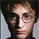2025 görüntülenme
This indicator calculates 3 Smoothed moving average for default values of
13, 8 and 5 days, with displacement 8, 5 and 3 days.
The most popular method of interpreting a moving average is to compare
the relationship between a moving average of the security's price with
the security's price itself (or between several moving averages).
```////////////////////////////////////////////////////////////
//  Copyright by HPotter v2.0 24/09/2014
// This indicator calculates 3 Smoothed moving average for default values of
// 13, 8 and 5 days, with displacement 8, 5 and 3 days: Median Price (High+Low/2).
// The most popular method of interpreting a moving average is to compare
// the relationship between a moving average of the security's price with
// the security's price itself (or between several moving averages).
////////////////////////////////////////////////////////////

study(title="Bill Williams Averages. 3Lines", shorttitle="3 Lines", overlay = true)
LLength = input(13, minval=1)
MLength = input(8,minval=1)
SLength = input(5,minval=1)
LOffset = input(8,minval=1)
MOffset = input(5,minval=1)
SOffset = input(3,minval=1)
SUM1 = sum(close, LLength)
SMMA1 = SUM1/LLength
PREVSUM1 = SMMA1 * LLength
SMMAL = (PREVSUM1-nz(SMMAL)+close)/LLength
SUM2 = sum(close, MLength)
SMMA2 = SUM2/MLength
PREVSUM2 = SMMA2 * MLength
SMMAM = (PREVSUM2-nz(SMMAM)+close)/MLength
SUM3 = sum(close, SLength)
SMMA3 = SUM3/SLength
PREVSUM3 = SMMA3 * SLength
SMMAS = (PREVSUM3-nz(SMMAS)+close)/SLength
plot(SMMAL[LOffset], color=blue, title="SMMA L")
plot(SMMAM[MOffset], color=red, title="SMMA M")
plot(SMMAS[SOffset], color=green, title="SMMA S")```

## YorumlarTHANK YOU so much for doing this.

Apparently they've added SMMA as an indicator. To test this, I compared a 13-period non-shifted SMMA (native indicator) to the shifted SMMA (your code here). They were very different. Perhaps it's possible to use the native SMMA method?
Cevap Gönderthe_batman
In the script I used formula from link what you send me in the other thread and code it. But I no not sure that is real formula. In the TV, how I know, don`t exists a builtin function of SMMA, but I can wrong.
Cevap Göndermoveon
Yeah, because the original is wrong. For the reason's stated in the original comment. (ie it uses SIMPLE moving average instead of SMOOTHED).
Cevap GönderHPotter
I think you're right. The formula I sent is wrong. (Sorry).

Here's the code (ie formula) from the SMMA indicator:

src = close
len = input(7, minval=1, title="Length")
smma = na(smma) ? sma(src, len) : (smma * (len - 1) + src) / len

Cevap Gönder